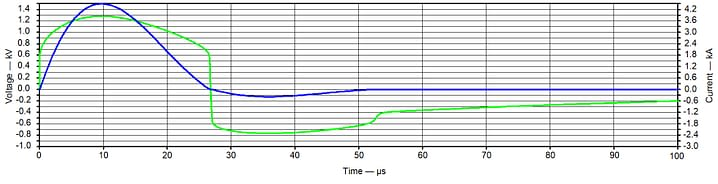# LTspice MOV simulationWith the knowledge that the logarithm of MOV resistance (R) has a reasonably linear relationship with the logarithm of MOV current (i), a function may be derived of the form LOG(R) =F(LOG(i)). In LTspice, this relationship allows the simulation of an MOV using a variable resistor controlled by R = 10^ F(LOG(i)), see Figure 5.

Three MOV circuit LTspice simulations are given; use with an 8/20 current generator (Figure 6), use with a 1.2/50-8/20 generator (Figure 11) and current sharing in paralleled MOVs (Figure 16).